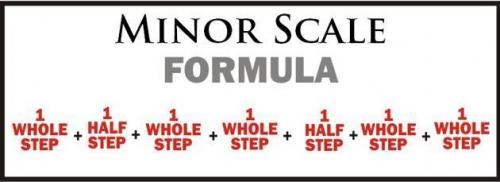## Minor Scales

The Minor Scale

The minor scale is also constructed of 8 pitches with the 1st and 8th pitch being the same letter name; however, the 1st and 8th pitch are 8 notes apart (octave).

Minor Scale Formula

Note: Whole step = 2 Half Steps

The formula is as followsThe shorthand the natural minor scale formula is

W-H-W-W-H-W-WThe harmonic minor scale is the same with 1 exception, the raised 7th scale degree.

W-H-W-W-H-(W+H)-H# Natural Minor Scales